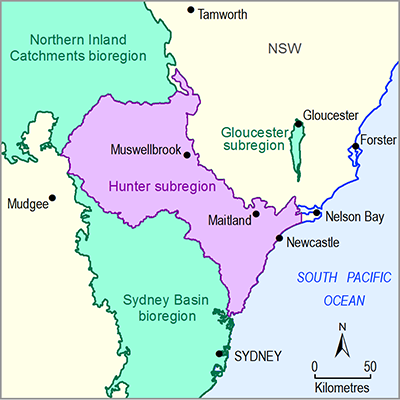# 2.1.5.2 Statistical analysis and interpolation

Page 27 of 41

## 2.1.5.2.1 Groundwater contributions to stream flow

A simple method to estimate the proportion of streamflow that is derived from groundwater is to assume that all recharge contributes to streamflow. As previously stated in Section 2.1.3.1, in the absence of other data, groundwater recharge is commonly estimated as 2% of rainfall (Mackie Environmental Research, 2006). For a mean annual rainfall of 793 mm for the subregion, an estimate of 16 mm of subregion mean annual recharge is obtained.

For steady-state conditions, and assuming all groundwater recharge discharges to the stream as baseflow, a crude estimate of the contribution from groundwater to streamflow can be made. The mean annual streamflow recorded in the Hunter River at Greta (gauge 210064) between 1968 and 2015 was 709.5 GL/year, equivalent to a depth of 41.0 mm across the 17,320 km2 contributing area (DPI, 2015). For 16 mm of recharge, this equates to a maximum baseflow contribution from groundwater of about 39%, with the remainder of Hunter River flow coming from surface and shallow subsurface runoff. The gauging station at Greta is used for this estimate because it is the most downstream gauge on the Hunter River that is not tidally influenced and represents the lower limit of the Hunter subregion river model.

Using the subregion estimate of mean annual recharge of 9.0 mm from the recharge surface reported in Section 2.1.3.2, this simple ratio approach to estimating the baseflow contribution to Hunter River flow at Greta (gauge 210064) yields a maximum baseflow contribution of 28%.

It is a simplification to assume that all diffuse recharge becomes river discharge even in a closed basin where evaporation and pumping occur (i.e. no other losses), however, this does provide an upper estimate for baseflow. It needs to be supported by other measures such as environmental tracers and computer models of mines near rivers. For example, in a groundwater modelling study at the North Wambo Underground Mine, fitted diffuse recharge was 1.2% of rainfall and alluvial discharge to the river accounted for two-thirds of all losses (Heritage Computing Pty Ltd, 2012). This could be used as a first estimate to partition aquifer discharge between baseflow and evaporation losses, reducing the previous maximum estimates of baseflow to 25.8 and 18.5%.

As previously described, the stream and alluvial aquifer are considered to be closely coupled and are managed conjunctively. Fluxes are expected in both directions under mean climatic conditions. In the case of modelling the North Wambo Underground Mine, the transient water balance estimated that from 2003 to 2009 the river leaked 12.1 ML/day into the alluvium and the alluvium returned 17.4 ML/day to the river. This is a net baseflow discharge of 5.3 ML/day, which based on the model area of 304 km2 equates to an annual depth of 6.4 mm.

It is difficult to make accurate generalisations of baseflow discharge for the subregion based on spatially averaged, or small-scale spatially explicit, models of recharge alone. If the total discharge values from the North Wambo model are representative of the subregion more generally this would equate to a gross discharge 20.9 mm (i.e. a baseflow index of 51.0%) or, taking account of the 12.1 ML/day that leaked from the river, a net baseflow contribution from groundwater of 15.3%.

To place this in context, estimates of baseflow index using digital filtering (Lyne and Hollick, 1979) vary from 40 to 66% for eight rivers in the Hunter river basin, and 44 to 49% for two rivers in the Macquarie Tuggerah Lakes basin (see Figure 44 in companion product 1.1 for the Hunter subregion (McVicar et al., 2015)). These estimates based solely on daily flow values do not account for leakage and return flow between the river and alluvium.

No other analyses were undertaken to inform the surface water and groundwater modelling. The foregoing estimates of baseflow contributions show that it is hard to specify this term for modelling purposes, but suggest an upper limit for the groundwater contribution to streamflow of 66%.

The modelling framework that has been developed represents surface water – groundwater interactions through using outputs from the groundwater model as inputs into the river model (see companion product 2.6.2 (groundwater numerical modelling) for the Hunter subregion (Herron et al., 2018)). For the purposes of constraining the modelled surface water – groundwater fluxes, it has been assumed that on average groundwater contributions to streamflow will not exceed 70% of the mean streamflow record (Herron et al., 2018). This proportion was deliberately chosen to be higher than the various estimates of baseflow that have been made for the Hunter subregion.

Last updated:
18 January 2019